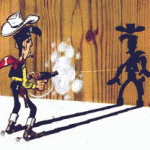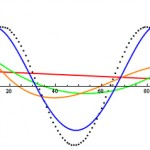# Posts Tagged ‘ Math ’

## Latent Semantic Indexing

March 7, 2011Here is a toy program that demonstrates LSI using the SVD contained within the COLT linear algebra library. The method is taken from here. import cern.colt.matrix.linalg.*; import cern.colt.matrix.*; import cern.colt.matrix.impl.*; import java.util.*; import java.io.*; public class SVDText { public static void main (String args[]) { try { DenseDoubleMatrix2D source = new DenseDoubleMatrix2D(3,11); Scanner sc = […]

Tags: ,
Posted in Uncategorized | Comments Off on Latent Semantic Indexing

## Curve Fitting via SVD

March 7, 2011I used this method to delete a gradient effect from uneven lighting of some of my book scans. Here is a simple method for curve fitting various polynomials. This works for non linear equations as long as the actual coeficients are linear. For example you can NOT fit the following type of function: Note that […]

Tags: ,
Posted in Uncategorized | Comments Off on Curve Fitting via SVD